# Periodic & Inverse Function Notes | EduRev

## JEE : Periodic & Inverse Function Notes | EduRev

The document Periodic & Inverse Function Notes | EduRev is a part of the JEE Course Mathematics (Maths) Class 12.
All you need of JEE at this link: JEE

Periodic Function
A function f(x) is called periodic if there exists a positive number T (T > 0) called the period of the function such that f (x + T) = f(x), for all values of x and x + T within the domain of f(x). The least positive period is called the principal or fundamental period of f.
Example: The function sin x & cos x both are periodic over 2π & tan x is periodic over π

Remark:
(a) A constant function is always periodic, with no fundamental period.
(b) If f(x) has a period p, then 1/f(x) and √f(x) also has a period p.
(c) If f(x) has a period T then f(ax + b) has a period  T/a  (a > 0).
(d) If f(x) has a period T1 & g(x) also has a period T2 then period of f(x) ± g(x) or f(x)/g(x) s L.C.M of T1 & Tprovided their L.C.M. exists. However that L.C.M. (if exists) need not to be fundamental period. If L.C.M. does not exists then f(x) ± g(x) or f(x). g(x) or f(x)/g(x) is non-periodic e.g. |sin x| has the period p, |cos x| also has the period π |sin x| + |cos x| also has a period p. But the fundamental period of |sin x| + |cos x| is π/2.
(e) If g is a function such that gof is defined on the domain of f and f is periodic with T, then gof is also periodic with T as one of its periods. Further if # g is one-one, then T is the period of gof # g is also periodic with T' as the period and the range of f is a subset of [0, T'], then T is the period of gof
(f) Inverse of a periodic function does not exist.

Example 1. Find period of the following functions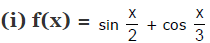(ii) f(x) = {x} + sin x
(iii) f(x) = cos x . cos 3x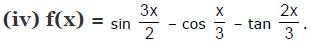Solution.
(i) Period of sin x/2 is 4p while period of cos x/3 is 6p. Hence period of sin x/2 + cos x/3 is 12 π {L.C.M. or 4 & 6 is 12}
(ii) Period of sin x = 2π ; Period of {x} = 1; but L.C.M. of 2π & 1 is not possible ∴ it is a periodic
(iii) f(x) = cos x . cos 3x ; Period of f(x) is L.C.M. of (2π, 2π/3) = 2π but 2π may or may not be the fundamental period. The fundamental period can be 2π/n where  n ∈ Np may or may not be the fundamental period. The fundamental period can be f(π + x) = (–cos x) (– cos 3x) = f(x)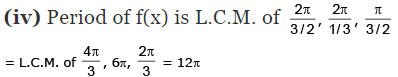Example 2. If f(x) = sin x + cos ax is a periodic function, show that a is a rational number.
Solution. Given f(x) = sin x + cos ax
Period of sin x = 2π/1  and period of cos ax 2π/a
Hence period of f(x) = L.C.M.
or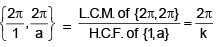where k = H.C.F. of 1 and a
1/k = integer = q (say), (≠0) and k a/k = integer = p (say)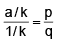a = p/q
a = rational number

Example 3. Given below is a partial graph of an even periodic function f whose period is 8. If [*] denotes greatest integer function then find the value of the expression.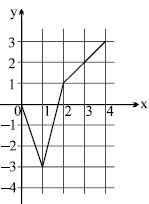f (-3) + 2 | f (-1) | + [f(7/8)] + f (0) + arc cos (f(-2)) + f (-7) + f (20)
Solution.
f (-3) = f (3) = 2 [f(x) is an even function, ∴ f(-x) = f (x)]
again f (-1) = f (1) = - 3
∴ 2 | f (-1) | = 2 | f (1) | = 2 | -3 | = 6
from the graph, -3 < f(7/8) < -3 ∴  [f(7/8)] = -3
f (0) = 0 (obviously from the graph)
cos -1 (f(-2)) = cos-1 (f (2)) = cos -1(1) = 0
f (-7) = f (-7 + 8) = f (1) = - 3 [f (x) has period 8]
f (20) = f (4 + 16) = f (4) = 3 [f (nT + X) = f (x)]
sum = 2 + 6 - 3 + 0 + 0 - 3 + 3 = 5

Example 4. If the periodic function f(x) satisfies the equation f(x + 1) + f(x - 1) = √3  f(x) ∀ x ∈ R then find the period of f(x)
Solution.
We have f(x + 1) + f(x – 1) = √3 f(x) ∀ x ∈ R
Replacing x by x – 1 and x + 1 in (1) then f(x) + f(x – 2) = √3 f(x – 1) ...(2)
Adding (2) and (3), we get 2f(x) + f(x – 2) + f(x + 2) = √3 (f (x – 1) + f(x + 1)) ...(3)
2f (x) + f(x – 2) + f(x + 2) = √3 . √3 f(x) [From (1)]
f(x + 2) + f(x – 2) = f(x) ...(4)
Replacing x by x + 2 in equation (4) then f (x + 4) + f (x) = f (x + 2) ...(5)
Adding equations (4) and (5), we get f(x + 4) + f (x - 2) = 0       ...(6)
Again replacing x by x + 6 in (6) then f (x + 10) + f (x + 4) = 0  ...(7)
Subtracting (6) from (7), we get f (x + 10) - f (x - 2) = 0     ...(8)
Replacing x by x + 2 in (8) then f (x + 12) - f(x) = 0 or f (x + 12) = f(x)
Hence f(x) is periodic function with period 12.

Inverse Of A Function
Let  f : A → B  be a one-one & onto function, then their exists a unique function
g :  B → A such that f(x) = y ⇔ g(y) = x, x ∈ A & y ∈ B. Then g is said to be inverse of f.
Thus g = f-1 :  B → A =  {(f(x), x) | (x,  f(x)) ∈ f}.

Properties of inverse function :
(i) The inverse of a bijection is unique, and it is also a bijection.
(ii) If f : A → B is a bijection & g : B → A is the inverse of f, then fog = IB and gof = IA, where IA & IB are identity functions on the sets A & B respectively.
(iii) The graphs of f & g are the mirror images of each other in the line y = x.
(iv) Normally points of intersection of f and f–1 lie on the straight line y = x. However it must be noted that f(x) and f–1(x) may intersect otherwise also.
(v) In general fog(x) and gof(x) are not equal. But if either f and g are inverse of each other or atleast one of f, g is an identity function, then gof = fog.
(vi) If f & g are two bijections  f : A → B ,  g : B → C then the inverse of gof exists and  (gof)-1 = f-1 og-1.

Example 5. Find the inverse of the function f(x) = ln(x2 + 3x +1); x ε [1, 3] and assuming it to be an onto function.
Solution.
Given f(x) = ln (x2 + 3x + 1)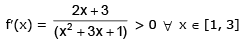which is a strictly increasing function. Thus f(x) is injective, given that f(x) is onto. Hence the given function f(x) is invertible. Now let y = f(x) = ln (x2 + 3x + 1) then x = f–1 (y) ...(1)
and y = ln (x2 + 3x + 1) ⇒ ey = x 2 + 3x + 1 ⇒ x2 + 3x + 1 – ey = 0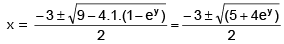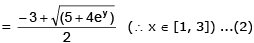From (1) and (2), we get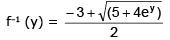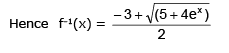Offer running on EduRev: Apply code STAYHOME200 to get INR 200 off on our premium plan EduRev Infinity!

## Mathematics (Maths) Class 12

209 videos|231 docs|124 tests

,

,

,

,

,

,

,

,

,

,

,

,

,

,

,

,

,

,

,

,

,

;